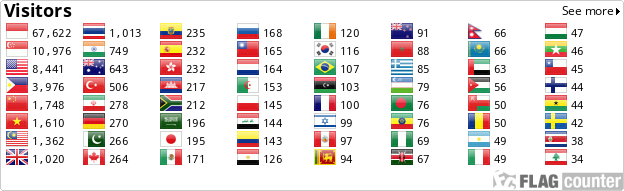### The Effect of Using SQ4R Technique on Students’ Reading Interest and Reading Comprehension at SMAN 1 Pangkalan Kuras Pelalawan

Ali Usman

#### Abstract

This research was carried out to find out the categories of students’ interest in reading and reading comprehension and the effect of using SQ4R Technique on their reading interest and reading comprehension at SMAN 1 Pangkalan Kuras Pelalawan. It was done based on a quasi-experimental design that requires a pre-test and post-test for a treated and comparison group. Sample of this research consisted of 76 students that were taken from two classes, namely Class XI IPS 1 as an experimental class (treated group) and Class XI IPS 2 as a control class (comparison group). Data were collected by using and questionnaire and reading comprehension test. SQ4R Technique was used as the treatment for the experimental group. The results indicated that there is a significant effect of implementing the treatment on students’ reading interest in the experimental group. T-test result was -3.4 and its df was 37, by comparing the significant value. If the probability value is > 0.05, the null hypothesis (H0) is accepted. If the probability value < 0.05, the alternative hypothesis (Ha) is accepted. Because the p-value was 0.002<0.05, thus, Ha is accepted while H0 is rejected. In addition, there is a significant effect of using SQ4R technique on the students’ reading comprehension. The t-test result was -6273 and its df was 37, by comparing the significant value. If the probability value is > 0.05, H0 is accepted and Ha is rejected. If the probability value is < 0.05, Ha is accepted and H0 is rejected. Because the p-value was 0.000 < 0.05, thus, Ha is accepted and H0 is rejected.

#### Full Text:

PDF

DOI: http://dx.doi.org/10.24014/ijielt.v5i2.12618

### Refbacks

• There are currently no refbacks.Indonesian Journal of Integrated English Language Teaching (p-ISSN 2355-5971)
Fakultas Tarbiyah dan Keguruan
Universitas Islam Negeri Sultan Syarif Kasim Riau

Indexed by :View My Stats